CAT  >  Base Systems

# Base Systems - Quantitative Aptitude (Quant) - CAT

 Table of contentsIntroductionConversions From One Base System to AnotherArithmetic Operations while Operating in a Specific Base System1. Addition2. Subtraction3. Multiplication4. DivisibilityImportant Concepts of Base SystemPrevious Year Questions based on Base System## Introduction

• The numbers that we commonly use are the decimal number. The system is called the decimal system. Why is it called the decimal system? It is because it has 10 symbols – 0, 1, 2, 3, 4, 5, 6, 7, 8, 9.
Example: 85 means – 8 * 101 + 5 * 100
• Here, 10 is known as the base of the decimal system.
• Base: It is the number of distinct symbols used in a particular number system.
So, depending on the number of digits in the base system, there are many other systems possible. Have a look at the following table: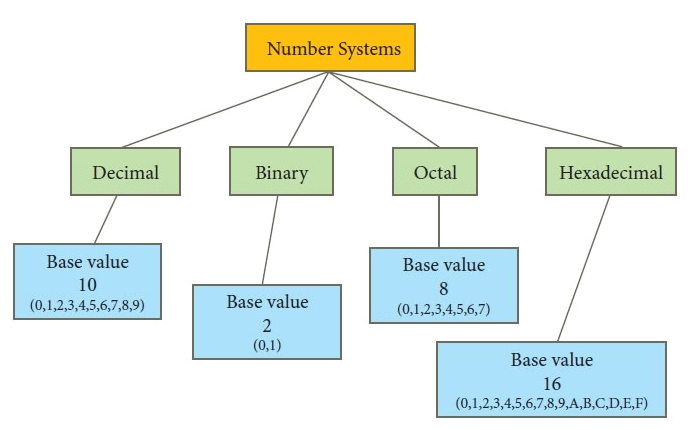Classification of Number System
• In the questions involving base systems, we are expected to figure out the different number of digits in that system of counting.
• Example: If we had 7 digits instead of 10 i.e. 0, 1, 2, 3, 4, 5, 6, we will have system of counting as follows: 0, 1, 2, 3, 4, 5, 6, 10, 11, 12, 13, 14… .
In above system , 45 will be expanded as = 4 * 91 +  5 * 90

## Conversions From One Base System to Another

### 1. Decimal to Binary

(a) (172)10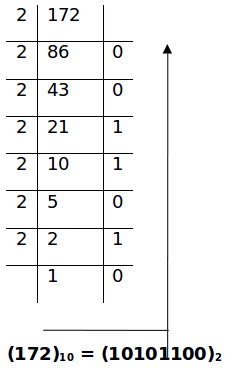(b) (28.3125)10
The given number has 2 parts:
(i) Conversion of an integral part: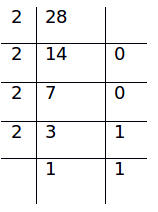(28)10 = (11100)2
(ii) Conversion of the fractional part:

• Multiply the decimal part with 2 successively and take the integral part of all the products starting from the first.
• 0.3125*2 = 0.6250 → (0)
• 0.6250*2 = 1.2500 → (1)
• 0.2500*2 = 0.500 → (0)
• 0.500*2 = 1 → (1)
• Therefore,
• (0.3125)10 = (0.0101)2
• (28.3125)10 = (11100.0101)2

Note: We should stop multiplying the factorial part by 2, once we get 0 as a fraction or the fractional part is non-terminating. It can be decided depending on the number of digits in the fractional part required.

### 2. Binary to Decimal

• (110101)2 = 1*25+ 1*24+ 0*23+ 1*22+ 0*21+ 1*20 = (53)10
• (0.11001)= 1*2-1+ 1*2-2+ 0*2-3+ 0*2-4+ 1*2-5 = (0.78125)10
Question for Base Systems
Try yourself:Convert the binary equivalent 10101 to its decimal equivalent.

### 3. Decimal to Octal

(a) (4324.235)10

(i) Integral Part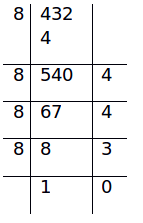(4324)10 = (10344)8

(ii) Fractional Part

• 0.235*8 = 1.88 → (1)
• 0.88*8 = 7.04 → (7)
• 0.04*8 = 0.32 → (0)
• We can stop here as the fraction is non-terminating.
• (4324.235)10 = (10344.170)8

Question for Base Systems
Try yourself:What could be the maximum value of a single digit in an octal number system?

### 4. Octal to Decimal

• (677.47)8
= 6*82+ 7*81+ 7*80+ 4*8-1+ 7*8-2 = (447.609375)10

• (23524)10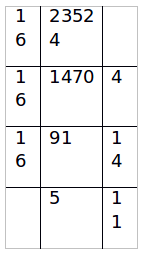(23524)10 = (5BE4)16

• (62A)16 = 6*162+ 2*161+ 10*160 = (1578)10
Now, we will look at conversions which do not include base 10. We can observe that the following cases include 2 bases (let them be a and b), where a=bn.

### 7. Binary to Octal

• (octal) is a cube of 2 (binary). So, to convert binary to octal, we just need to club three digits of binary number starting from unit digit and write the decimal equivalent of each group.
• (1101001011)2 = (001 101 001 011)2
(We can introduce two zeroes to form groups of 3 without changing the magnitude of the number)
(001 101 001 011)= (1513)8

### 8. Octal to Binary

Here, we need to express every digit of octal number into its binary form comprising of 3 digits.

• (645)8
• 6 = (110)2
• 4 = (100)2
• 5 = (101)2
• (645)8 = (110 100 101)2

### 9. Binary to Hexa-Decimal

• It is similar to the method discussed in the 7th point. Instead of clubbing 3, we club 4 digits.
• (10101110)2 = (1010 1110)2 = (AE)16

### 10. Hexa-Decimal to Binary

• We need to express every digit of Hexa-decimal into its binary form comprising of 4 digits.
• (3A91)16
• 3 = (0011)2
• A = (1010)2
• 9 = (1001)2
• 1 = (0001)2
• (3A91)16 = (11101010010001)2

Note: Using the logic discussed in points 7 to 10, we can do direct conversions in any two bases a and b such that a=bn where we will form blocks of n digits when the number is in base b and then write its decimal equivalent.

Example: For conversion from base 3 to base 9, we need to make blocks of 2 digits as 9 = 32, for instance- (22112)3 = (02 21 12)3 = (275)9

## Arithmetic Operations while Operating in a Specific Base System

• One method of performing arithmetic operations is to first convert the numbers to the decimal system, perform the required operations and then convert the numbers back to the required base.
• However, arithmetic operations can also be directly done given that numbers are expressed in the same base.

(a) (8358)10 + (5684)10

(8358)10 + (5684)10 = (14042)10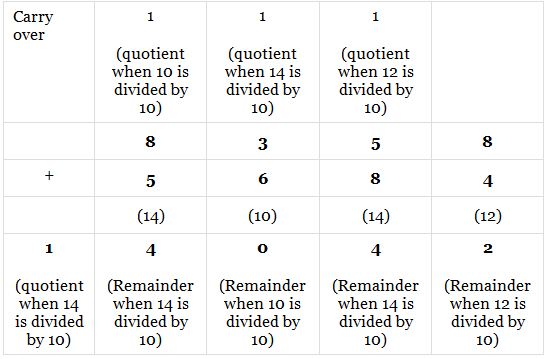➢ Logic
• Start from a unit position; 8+4=12, and 12, when divided by 10 (base), gives us remainder as 2, which is the total for that column. 12, when divided by 10, gives us quotient as 1, which is carried over to the next column.
• In the second column, 1+5+8=14. When 14 is divided by 10 (base), we get 4 as remainder (total for that column) and 1 as quotient which is carried over to next column.
• In third column, 1 + 3 + 6 = 10. 10, when divided by 10, gives us 0 as remainder and 1 as quotient. We proceed in similar and get the required sum.
(b) (3542)6 + (4124)6

###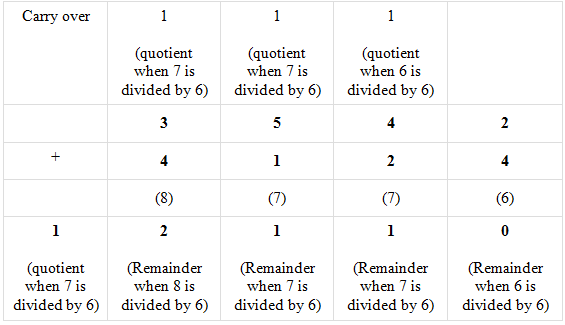(3542)+ (4124)6 = (12110)6

## 2. Subtraction

(a) (237)10 – (199)10

(237)10 – (199)10 = (38)10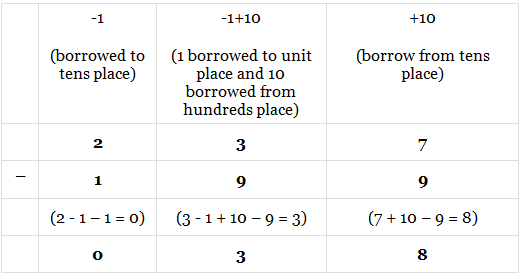➢ Logic
• In units place 7 < 9, hence we borrow 10 (base) from tens place. 17-9=8
• Tens place reduces from 3 to 2. Still 2<9, hence we borrow 10 (base) from hundreds place. 12-9=3
• Hundreds place reduces to 1 (as we borrowed from it in an earlier step). 1-1=0
(b) (422)5 – (243)5

###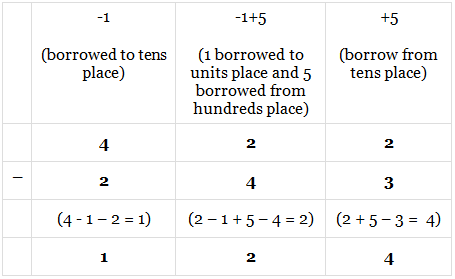(422)5 – (243)5 = (124)5

## 3. Multiplication

Using the analogy of base 10, we can multiply in other bases too.

(a) (346)7 * (4)7

###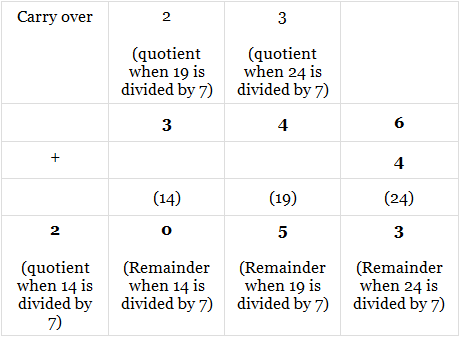(346)7 * (4)7 = (2053)7

(b) (76)8 * (45)8

###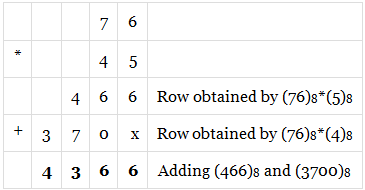(76)8 * (45)8 = (4366)8

## 4. Divisibility

(a) Is (7364)divisible by 8?

• The logic behind this question is same as checking the divisibility of any number (in decimal system) by 9. We add the digits and then check the divisibility.
• 7 + 3 + 6 + 4 = 20 which is not divisible by 8. Hence, the given number is not divisible by 8.

Rule: (x)b is divisible by (b-1) if all the digits of (x)b add up to be divisible by (b-1).

(b) Is (5236)9 divisible by 10?

• The logic behind this question is same as checking the divisibility of any number (in decimal system) by 11.
• We first find the sum of alternate digits and then find the difference of the sums obtained. This difference should either be divisible by 0 or divisible by 11(or 10 in the case of this question).
• 5 + 3 = 8; 2 + 6 = 8
• 8 - 8 = 0. Hence, the number is divisible by 10.

Rule: (x)b is divisible by (b+1) if the difference of the sums of alternate digits of (x)b is either 0 or divisible by (b+1).

(c) What is the IGP (Index of Greatest Power) of 9 in (780)9?

• (780)9 = 7*92+ 8*91+ 0*90 = 9(7*9+ 8)
• Thus IGP= 1. As the highest power of 9 with which the number is divisible is 1.

Rule: For a number in base b, if there are k zeroes in the end then it is divisible by bk. Also, k is the IGP of b in the number.

## Important Concepts of Base System

Example 1. Find the fifth root of (15AA51)19

• (15AA51)19 = 1*195+ 5*194+ 10*193+ 10*192+ 5*191+ 1*19= (19+1)5 = 20(Using binomial theorem)
• Therefore, the fifth root is 20.

Other examples of similar kind are:

• (121)n = n2+ 2n+ 1= (n+1)(n>2)
• (1331)= n3+ 3n2+ 3n+ 1= (n+1)3 (n>3)
• (14641)n, (15AA51)n and so on (the digits of the numbers used in the above examples form Pascal’s triangle)

Example 2. How many 4-digit numbers in base 9 are perfect squares?

• First, we need to know the range of 4-digit numbers in base 9
• Least 4 digit number possible= (1000)= 93 =729

• Observation: Lowest n digit number in base k = k(n-1)
• Highest 4 digit number possible= (8888)9 = 94-1= 6560

• Observation: Highest n digit number in base k = kn-1
• From 729 to 6560, the squares vary from 272 to 802.
• Number of perfect squares present = 80 - 26 = 54.

## Previous Year Questions based on Base System

Q.1. Let a, b, c be distinct digits. Consider a two-digit number ‘ab’ and a three-digit number ‘ccb’, both defined under the usual decimal number system, if (ab)2 = ccb > 300, then the value of b is? (CAT 1999)
(a) 1
(b) 0
(c) 5
(d) 6

• (ab)2 = ccb, the greatest possible value of ‘ab’ can be 31, since 312 = 961 (and since ccb > 300), 300 < ccb < 961, so 18 < ab < 31.
• So the possible value of ab which satisfies (ab)2 = ccb is 21.
• So 212 = 441, a = 2, b = 1, c = 4.

Q.2. Convert the number 1982 from base 10 to base 12. The result is? (CAT 2000)
(a) 1182
(b) 1912
(c) 1192
(d) 1292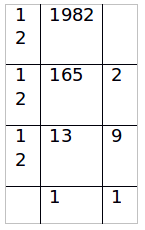Q.3. In a number system, the product of 44 and 11 is 1034. The number 3111 of this system, when converted to the decimal number system, becomes? (XAT 2001)
(a) 406
(b) 1086
(c) 213
(d) 691
(e) None of the above

• Let the base be n
• (4n+4)(n+1) = n3+3n+4
• n3-4n2-5n = 0
• n(n-5)(n+1) = 0
• n = 5
• (3111)= (406)10

Q.4.The product of two numbers 231 and ABA is BA4AA in a certain base system (where the base is less than 10), where A and B are distinct digits. What is the base of that system? (CAT 2010)
(a) 5
(b) 6
(c) 7
(d) 8
(e) 4

• Hence ,we can write (b+4+a)-2a = 0 or (base)+ 1 (let’s take (base+1))
i.e. b = base + a – 3

• 231 * aba = 2a(base)4+(2b+3a)(base)3+(3a+3b)(base)2+(3a+b)(base)+a
• Now put b = base + a – 3 , in above equation and Compare it with ba4aa, We get:
• 2a + 2 = b
• 4a – 3 = a
• Solving them gives a = 1 , b = 4
• Hence, base = b - a + 3 = 6
The document Base Systems | Quantitative Aptitude (Quant) - CAT is a part of the CAT Course Quantitative Aptitude (Quant).
All you need of CAT at this link: CAT

## Quantitative Aptitude (Quant)

179 videos|155 docs|113 tests

## FAQs on Base Systems - Quantitative Aptitude (Quant) - CAT

 1. What are base systems?Ans. Base systems are mathematical systems that use a specific number of digits to represent all numbers. The most commonly used base system is the decimal system, which uses ten digits (0-9). Other base systems include binary (base-2), octal (base-8), and hexadecimal (base-16).
 2. How do you convert numbers from one base system to another?Ans. To convert a number from one base system to another, you need to first convert it to decimal form and then convert it to the desired base system. For example, to convert a number from binary to decimal, you multiply each digit by its corresponding power of 2 and add up the results. Then, to convert the decimal number to octal, you divide it by 8 and write down the remainder for each division, starting from the bottom.
 3. What are the arithmetic operations that can be performed in a specific base system?Ans. The arithmetic operations that can be performed in a specific base system include addition, subtraction, multiplication, and divisibility. To perform these operations, you need to follow the rules of the specific base system, such as carrying over or borrowing digits when necessary.
 4. What are the important concepts of base system?Ans. The important concepts of base systems include place value, digit value, and positional notation. Place value refers to the value of a digit based on its position in a number, while digit value refers to the numerical value of a digit in a specific base system. Positional notation is the system of writing numbers using digits in specific positions to indicate their place value.
 5. Can you provide an example of a previous year question based on base system?Ans. Q: Convert the hexadecimal number 3B7A to binary. (from the GATE 2019 exam) A: To convert the hexadecimal number 3B7A to binary, you can first convert it to decimal form using the formula 3*16^3 + 11*16^2 + 7*16^1 + 10*16^0 = 15258. Then, you can convert the decimal number to binary by dividing it by 2 and writing down the remainder for each division, starting from the bottom. The binary equivalent of 15258 is 11101110111010.

## Quantitative Aptitude (Quant)

179 videos|155 docs|113 testsExplore Courses for CAT exam### How to Prepare for CAT

Read our guide to prepare for CAT which is created by Toppers & the best Teachers
Signup to see your scores go up within 7 days! Learn & Practice with 1000+ FREE Notes, Videos & Tests.
10M+ students study on EduRev
Track your progress, build streaks, highlight & save important lessons and more!
Related Searches

,

,

,

,

,

,

,

,

,

,

,

,

,

,

,

,

,

,

,

,

,

;